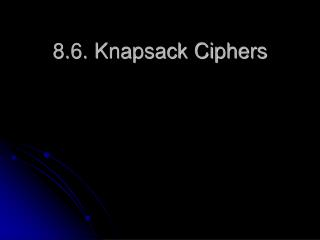DownloadDownload Presentation8.6. Knapsack Ciphers

8.6. Knapsack Ciphers

Download Presentation8.6. Knapsack Ciphers

- - - - - - - - - - - - - - - - - - - - - - - - - - - E N D - - - - - - - - - - - - - - - - - - - - - - - - - - -
Presentation Transcript

1. 8.6. Knapsack Ciphers

2. The Concept • At the core of the Knapsack cipher is the Knapsack problem: Given positive integers a1, a2,…,an & S, which of the ai integers add up to S. • As an equation, solve for xieither 1 or 0: S = a1 x1 + a2 x2 + … + an xn • Example: a1 = 2, a2=3, a3=4, a4=7, a5=11, a6=13, a7=16 and S=18 • Solutions: 16+2, 13+3+2, 11+4+3, 11+7, thus • (1,0,0,0,0,0,1); (1,1,0,0,0,1,0); (0,1,1,0,1,0,0); (0,0,0,1,1,0,0)

3. S = a1 x1 + a2 x2 + … + anxncomplexity Good vs. Bad • Difficult Calculations when n is large: Trial and Error  2n possibilities for (x1,x2,…,xn)  infeasible to find all the solutions when n=100 or more • Easier to find solution for certain a1, a2,…,an: aj=2J-1 S = a1 x1 + a2 x2 + … + an xn = 20 x1 + 21 x2 + … + 2n-1 xn = xn … x2 x1on binary form (base 2) Thus for the solution is: write S in the binary form!

4. Super Increasing Sequences • A type of sequence a1, a2,…,an for which it is easier but not trivial to solve knapsack problems • super increasing sequence if jth term > sum of the preceding values Σk(j-1) ak < aj forj = 2,3,…,n • Example1: (2, 3, 7, 13, 28) is super increasing (2, 3, 4, 7, 11, 13, 16) is not • Example2 (pb 3): aj+1 > 2aj super increasing sequence • Example3 (pb 2): aj < 2j-1 NOT super increasing sequence

5. Example of solving Knapsack problem for super increasing sequence • (a1=2, a2=3, a3=7, a4=13, a5=28) and S=40 • S≥ a5 x5 = 1 since a1+a2+a3+a4< a5=28 • S- x5 a5 = 12 < a4 =13  x4 =0 • S- (x5 a5 + x4a4 )=12 ≥ a3 x3 = 1 • S- (x5 a5 + x4a4+ x3a3 )=5 ≥ a2 x2 = 1 • S- (x5 a5 + x4a4+x3a3 + x3a3 )=2 ≥ a1 x1 = 1 • Solution: (1,1,1,0,1)

6. Super Increasing Algorithm • S = a1 x1 + a2 x2 + … + an xn

7. Public cryptosystem: Knapsack Ciphers based on super increasing sequences • Merkle and Hellman [MeHe78]. • Based on a transformed not super increasing sequence b1, b2,…,bn from a simple super increasing a1, a2,…,an Given m> 2 anand(ω,m)=1 findώ ώ ω ≡1(mod m)  ώ ≡ ωΦ(m)-1 (mod m) Then Find bj bj ≡ ω aj (mod m) aj ≡ ώ bj (mod m)

8. Observations • IF S= b1 x1 + b2 x2 + … + bn xnThen ώ S ≡ ώ b1x1 + …+ ώ bnxn ≡ a1x1 + …+ anxn(mod m) • b1, b2,…,bn is not super increasing  not easy to solve S= b1 x1 + b2 x2 + … + bn xn • a1, a2,…,an is super increasing  easy to solve S0= a1 x1 + a2 x2 + … + an xn whereώ S ≡ S0(mod m) • One needs to know m, ω & ώ

9. Knapsack Cipher Method • Choose a1, a2,…,aN along with values for m with m>2aN, and ω with (ω, m)=1 • (b1,…, bN) is made public. • Plaintext P is transformed into binary equivalent using the table on the left (page 319). • P in binary is split into segments of length N (if not divisible by N, add 1s) • Each segment will play the role of (x1,x2,…,xN)

10. Knapsack Cipher Method (cont.) • For each segment (x1,x2,…,xN) in P, compute S= b1 x1 + b2 x2 + … + bN xN • C= Ciphertext = the set of S generated from each (x1,x2,…,xN) in P = difficult to find (x1,x2,…,xN) from S • Decryption when m & ω (thus ώ) are known:  easy to solve for (x1,x2,…,xN) with S0= a1 x1 + a2 x2 + … + an xn whereώ S ≡ S0(mod m)

11. Example • P=BUY NOW=000011010011000011010111010110 • A=(3,5,9,20,44); m=89; ω=67 • P= ([0,0,0,0,1],[1,0,1,0,0],[1,1,0,0,0],[0,1,1,0,1],[0,1,1,1,0],[1,0,1,1,0]) = matrix notation Encryption (BT = transpose of B = Vertical vector B) • B≡ ω A (mod 89) = (23,68,69,5,11) • C= PBT = (11, 92,91,148,142,97 ) Decryption: • ώ ≡ ωΦ(m)-1 (mod m) =6787= 4 (mod 89) • S0≡ ώ S (mod m) ≡ 4*C = (44, 368, 364, 592, 568, 388) ≡ [44, 12, 8, 58, 34, 32] (mod 89) • Use Knapsack algorithm to solve S0= a1 x1 + a2 x2 + … + an xn • For example: 44= 44(1)  00001  B 12=9 (1) + 3(1) 10100  U 8= 3(1)+ 5 (1)  11000  Y

12. Cryptanalysis • Knapsack Ciphers were a popular form of public key cryptography. • In 1982, Shamir (see [Sh84] & [Od 90])  efficient method to solve S= b1 x1 + b2 x2 + … + bN xN, thus find x1x2…xNfrom the transformed public key b1b2 …bN • There exists an algorithmto find the solution using O(P(n)) bit operations where P is a polynomial instead of the exponential time • Adjustments can be made to protect it from such weaknesses, such as using several successive transformations with (ωi,mi) to form b1b2 …bN